# Lebesgue constants

(diff) ← Older revision | Latest revision (diff) | Newer revision → (diff)

The quantitieswhere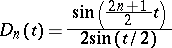is the Dirichlet kernel. The Lebesgue constants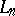for eachequal:

1) the maximum value offor alland all continuous functionssuch thatfor almost-all;

2) the least upper bound offor alland all continuous functionssuch that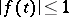;

3) the least upper bound of the integrals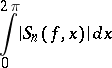for all functionssuch that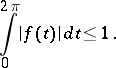Here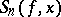is the-th partial sum of the trigonometric Fourier series of the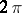-periodic function. The following asymptotic formula is valid: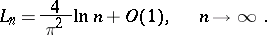In particular,as; this is connected with the divergence of the trigonometric Fourier series of certain continuous functions. In a wider sense the Lebesgue constants are defined for other orthonormal systems (cf. Orthogonal system) as the quantitieswhere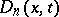is the Dirichlet kernel for the given orthonormal system of functions on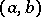; they play an important role in questions of convergence of Fourier series in these systems. The Lebesgue constants were introduced by H. Lebesgue (1909). See also Lebesgue function.

## Contents

How to Cite This Entry:
Lebesgue constants. Encyclopedia of Mathematics. URL: http://encyclopediaofmath.org/index.php?title=Lebesgue_constants&oldid=12712
This article was adapted from an original article by K.I. Oskolkov (originator), which appeared in Encyclopedia of Mathematics - ISBN 1402006098. See original article